Number Visualization

math art kotlin

Simple algorithms to visualize numbers or sequences.

The general form of the algorithms is to walk a sequence of digits and move a cursor up, down, left, right, or diagonal. As the cursor moves it draws a pixel who's color is determined by other means.

Codebase can be found on GitHub

π

100k digits, base 4, start from most significant bit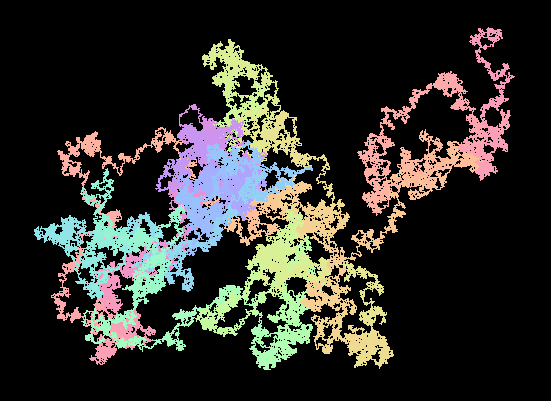100k digits, base 4, start from least significant bit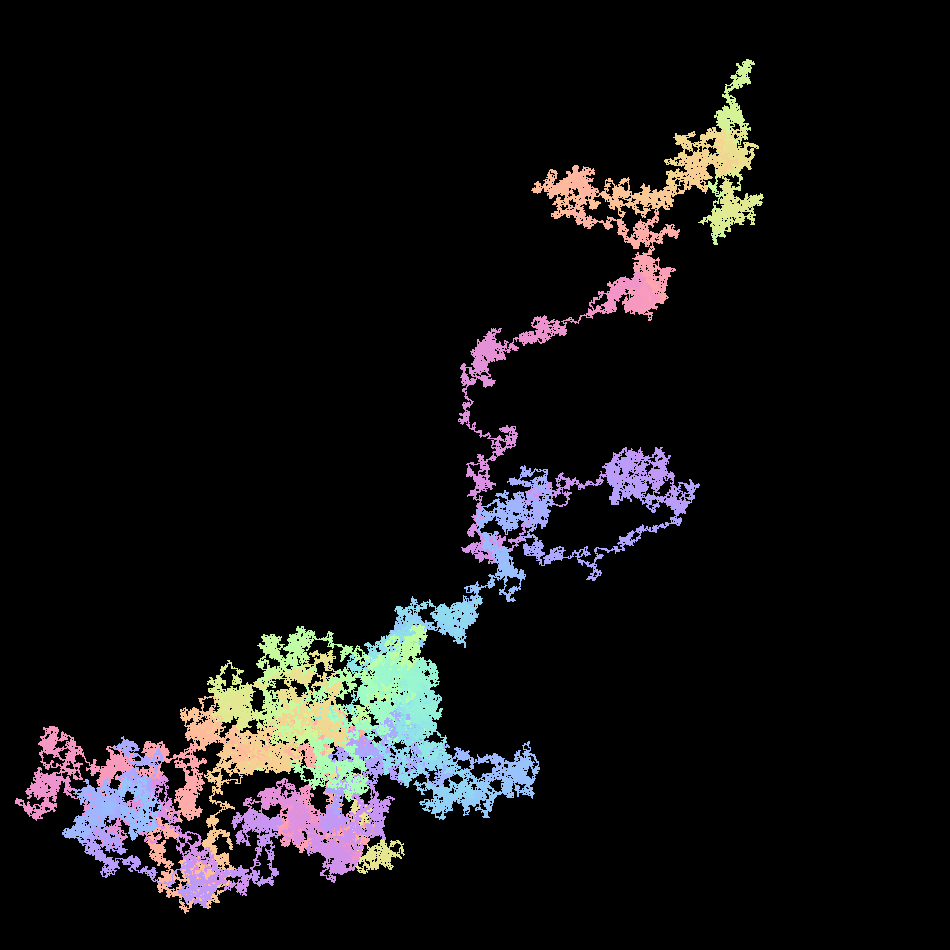e

1,000,000 digits, base 4, start form most significant bit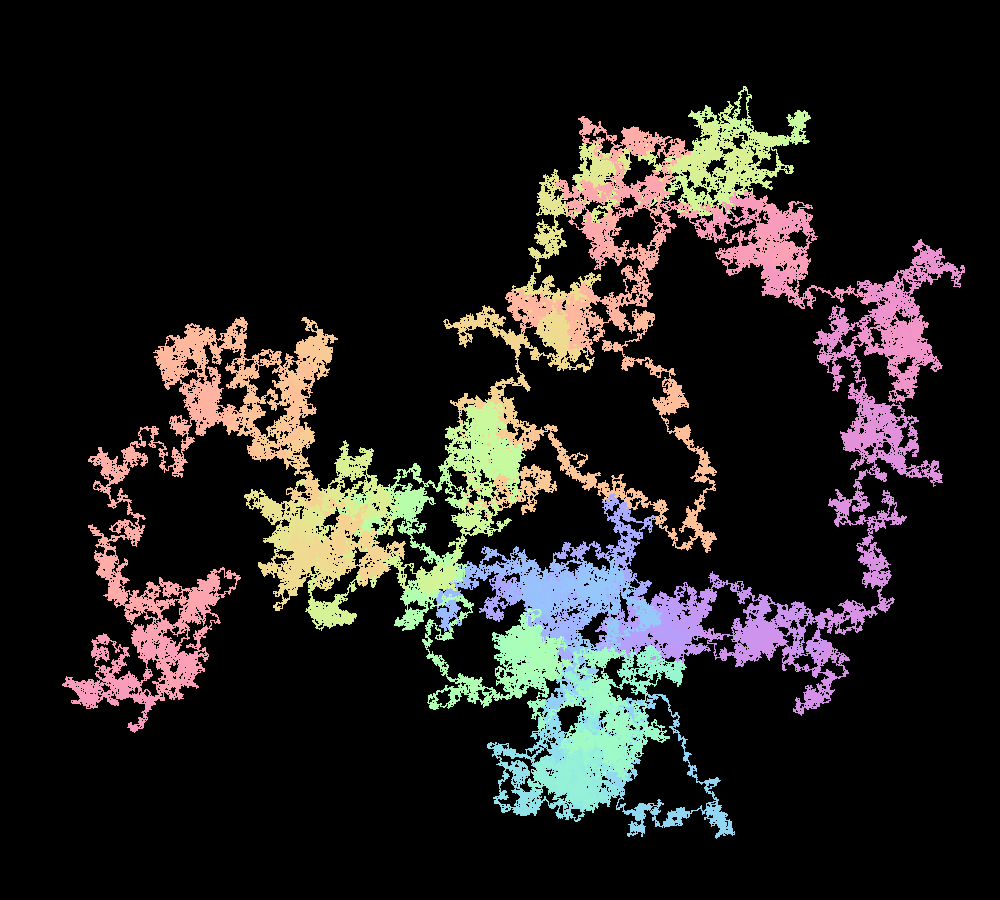1,000,000 digits, base 4, start from least significant bit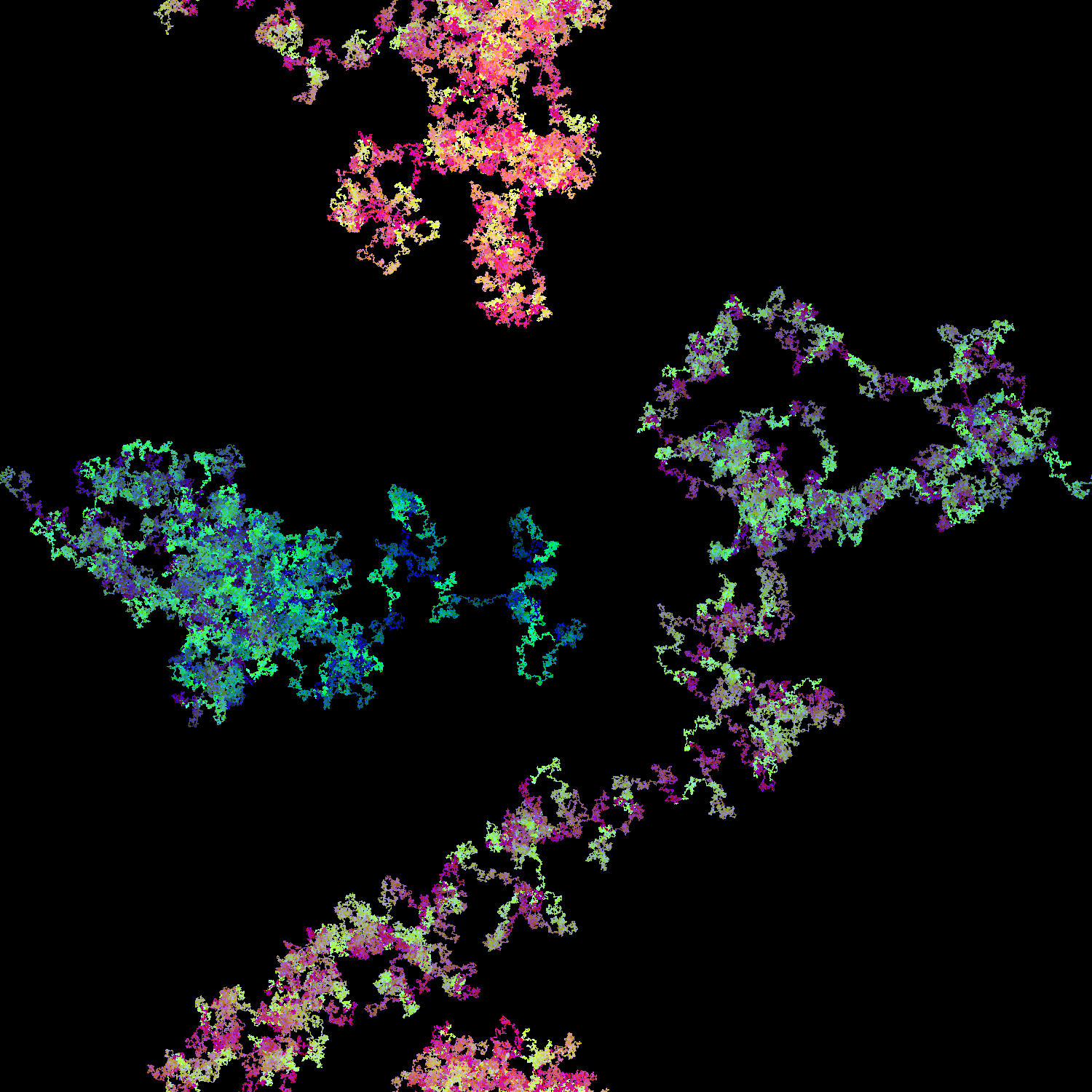1,000,000 digits, base 4, start from least significant bit, fill in space with decreasing color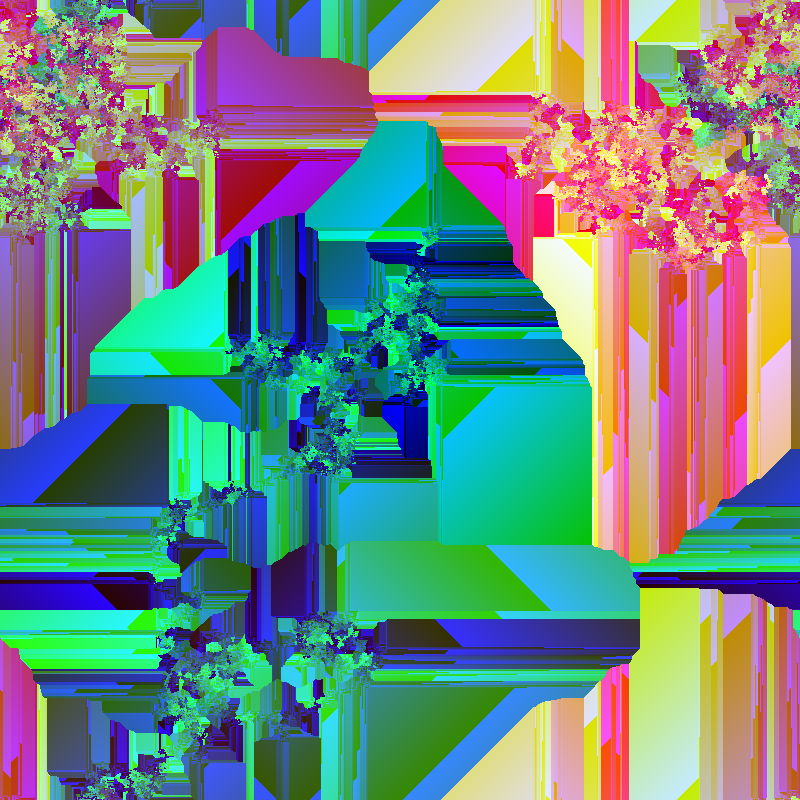Mersenne 35 (2^1,398,269 - 1)

base 10, 8 directions, start from most significant bit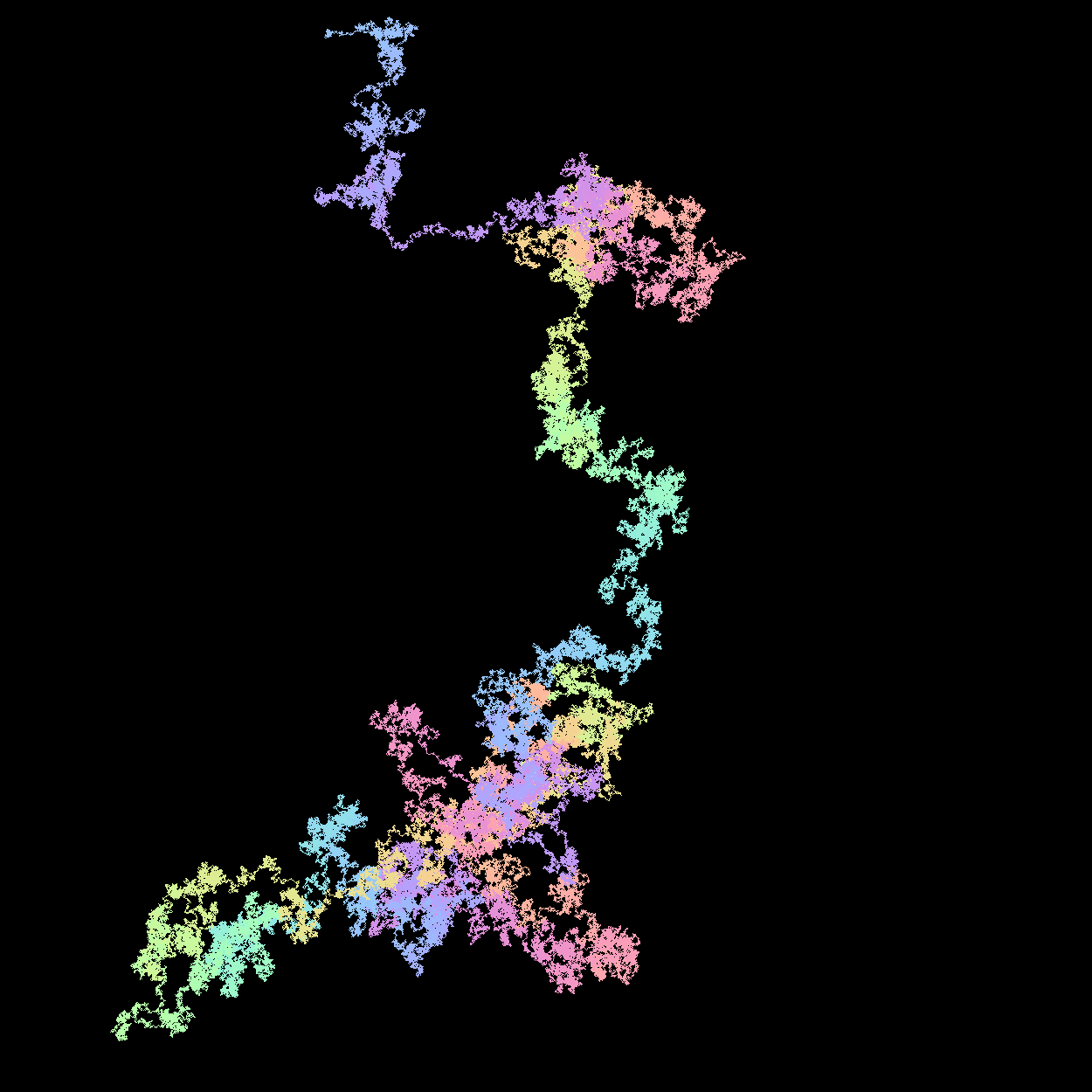Misc Numbers & Sequences

base 4, random digits (0-3)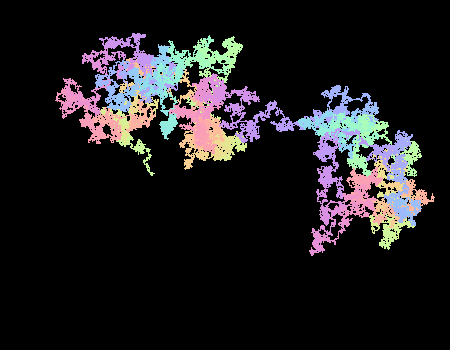To determine the color of the current pixel a value is stored in each (x,y) space equal to iteration/max_iteration * 0xFFFFFF.

The value can be mapped directly to an RGB. However, this does not result in a smooth color gradient. To generate a smooth gradient, I instead treat that value as an input into another function that "walks around" the color wheel (that you may see in many art apps). This results in a smoother color transition. More can be understood by reading this post. This post provides a very good starting point for understanding color gradients.

In code the color function is shown below. i in this case is the ith element in the sequence being plotted to the grid.

class SmoothColorizer(private val f1: Double = 0.3,
private val f2: Double = 0.3,
private val f3: Double = 0.3,
private val p1: Double = 0.0,
private val p2: Double = 2.0,
private val p3: Double = 4.0,
private val center: Int = 128,
private val width: Int = 127) : Colorizer {

override fun apply(i: Int): Color {
val r = Math.sin(f1 * i + p1) * width + center
val g = Math.sin(f2 * i + p2) * width + center
val b = Math.sin(f3 * i + p3) * width + center

return Color(r.toInt(), g.toInt(), b.toInt())
}
}